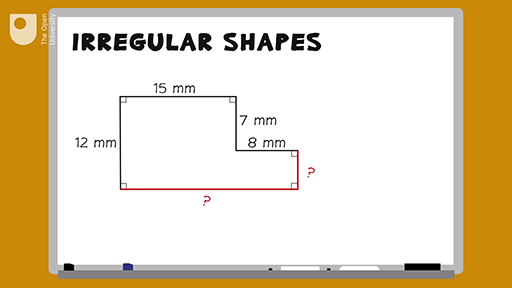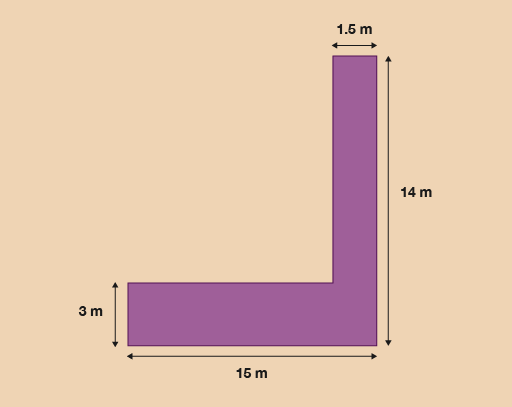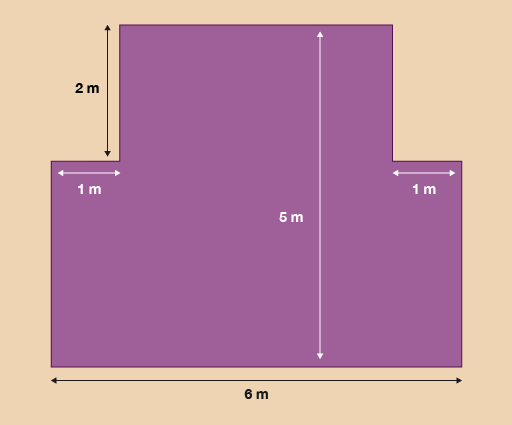Science, Maths & Technology

### Become an OU studentEveryday maths 1

Start this free course now. Just create an account and sign in. Enrol and complete the course for a free statement of participation or digital badge if available.

# 1.1 Measuring the perimeter of irregular shapes

## Example: How to measure the perimeter of an irregular shape

How would you measure the perimeter of an irregular shape – an L-shaped room, for instance – if you didn’t have all of the measurements that you would need? Watch the following video to find out.Interactive feature not available in single page view (see it in standard view).

Now try the following activity. Remember to check your answers once you have completed the questions.

## Activity 3: Finding the perimeter

Note that you can assume that all of the corners in the images in this activity are right angles.

1. A gardener decides to lay a new path next to his lily pond. The drawing shows the dimensions of the path.Figure 6 A pathway

The gardener decides to paint a white line around the perimeter of the path. What is the perimeter of the path?

2. A tourist information centre has a new extension.Figure 7 A new extension

The tourist board wants to attach a gold strip around the border of the floor of the building. What is the perimeter of the new extension?

1. To calculate the missing sides you should have carried out the following calculations:

• 14 – 3 = 11
• 15 – 1.5 = 13.5

Now that you have found the missing sides, you can add them all together:

• 15 + 14 + 1.5 + 3 + 13.5 + 11= 58 m
2. To calculate the missing sides you need to carry out the following calculations to calculate the perimeter:

• 5 – 2 = 3
• 6 – 2 = 4

Now that you have found the missing sides, you can add them all up together to calculate the perimeter:

• 6 + 3 + 3 + 1 + 1 + 2 + 2 + 4 = 22 m

## Summary

In this section you have learned how to work out the perimeter of both simple and irregular shapes.